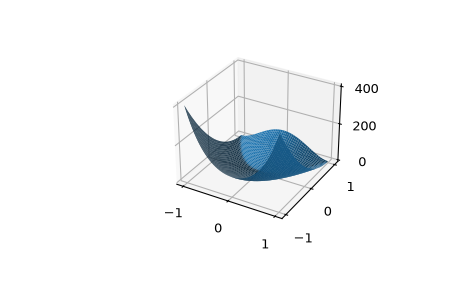# scipy.optimize.rosen¶

scipy.optimize.rosen(x)[source]

The Rosenbrock function.

The function computed is:

sum(100.0*(x[1:] - x[:-1]**2.0)**2.0 + (1 - x[:-1])**2.0)

Parameters
xarray_like

1-D array of points at which the Rosenbrock function is to be computed.

Returns
ffloat

The value of the Rosenbrock function.

Examples

>>> from scipy.optimize import rosen
>>> X = 0.1 * np.arange(10)
>>> rosen(X)
76.56


For higher-dimensional input rosen broadcasts. In the following example, we use this to plot a 2D landscape. Note that rosen_hess does not broadcast in this manner.

>>> import matplotlib.pyplot as plt
>>> from mpl_toolkits.mplot3d import Axes3D
>>> x = np.linspace(-1, 1, 50)
>>> X, Y = np.meshgrid(x, x)
>>> ax = plt.subplot(111, projection='3d')
>>> ax.plot_surface(X, Y, rosen([X, Y]))
>>> plt.show()